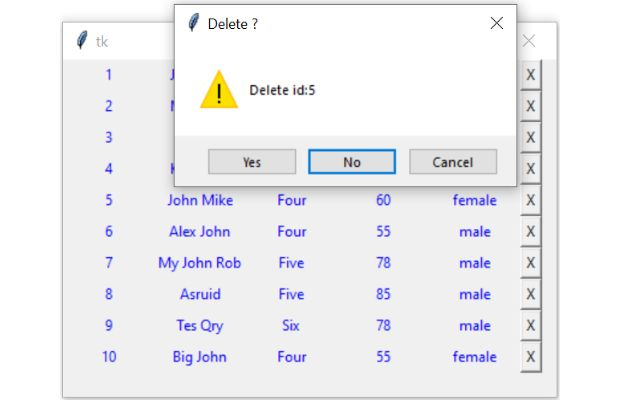# Delete record on button click

Basics of Python Tkinter

Display records from sqlite Student table in Tkinter window. Each row will have one button and on click of the button one message box will appear to confirm the user choice. User can confirm or cancel the delete operation.## Connect to sqlite / MySQL database

We connected to sqlite database and create our student table with sample data.
``````import sqlite3
my_conn = sqlite3.connect('my_db.db')``````
We will use my_conn in our further script as the connection object to get our records.

The script is same so it will work without any issue with both SQLite and MySQL database. Here is the connection string with MySQL database. Use the SQL dump from here to create tables
``````from sqlalchemy import create_engine
We used Query with LIMIT to collect 10 records and displayed in our window.

## Display rows with delete button

By using LIMIT Query we are restricting our number of records by getting 10 records only from SQLite / MySQL database. You can change this figure to get different number of records from the database.

Note that SQLite / MySQL database executes the query part only and return us one result set. This result set contains all our (10) records.
We used the returned record set i.e r_set as an iterator.

We will use one for loop to collect each row of record from the data set. Each row of data ( here student ) is a tuple. So we used another for loop to display each element of this tuple.

At the end of each row we will keep one Delete button marked with 'X' to delete the record. On click of this record we will pass the unique ID of the row to my_delete() function.

We kept the these code inside a function my_show() as we can call this function to take a fresh copy of records after executing the delete command.

Inside the function first we are removing the previously displayed rows by using grid_slaves().
``````def my_show():
for w in my_w.grid_slaves(): # remove all rows first
w.grid_forget() # remove row

r_set=my_conn.execute('''SELECT * from student LIMIT 0,10''');
i=0 # row value inside the loop
for student in r_set:
for j in range(len(student)):
e = Entry(my_w, width=10, fg='blue')
e.grid(row=i, column=j)
e.insert(END, student[j])
e = Button(my_w, text='X',command=lambda d=student : my_delete(d))
e.grid(row=i, column=j+1)
i=i+1``````
This will print 10 rows of records from student table and each row will have one Delete button at the end. The click event of the button will pass the unique row id to the function my_delete().

## Using tkinter

We will use one tkinter entry component to display each data in the window. In this variable student is a tuple and it contains one row of data. We used variable i as index for each row and variable j as each column of data.

## Delete the record

Each button at the end will carry the row id or student id. On click of this button our my_delete() function will get the id value.
``e = Button(my_w, text='X',command=lambda d=student : my_delete(d)) ``
To understand how to create buttons dynamically and use the click event to pass value click here.
``````def my_delete(id):
if my_var: # True if yes button is clicked
r_set=my_conn.execute("DELETE FROM student WHERE id=" + str(id) );
msg.showerror("Deleted ","No of records deleted = " + str(r_set.rowcount))
my_conn.commit()
my_show() # refresh the window with new records``````
Our function my_delete(id) receives value of id and display one message box with option asking user to confirm. User can select Yes, No or cancel. Our variable my_var will receive the user choice. One if condition is used to check the value of my_var and if it is True then delete query is executed.

After deleting, the number of records deleted is shown by using rowcount() using one message box. We have again called the my_show() function to take a fresh copy of records as one record is deleted.
The full code is here.
``````# www.plus2net.com
import sqlite3
my_path="D:\\testing\\sqlite\\my_db.db" #Change the path
my_conn = sqlite3.connect(my_path)
###### end of connection ####

##### tkinter window ######
import tkinter  as tk
from tkinter import *
from tkinter import messagebox as msg
my_w = tk.Tk()
my_w.geometry("400x350")
def my_show():
for w in my_w.grid_slaves(): # remove all rows first
w.grid_forget() # remove row
r_set=my_conn.execute('''SELECT * from student LIMIT 22,10''');
i=0 # row value inside the loop
for student in r_set:
for j in range(len(student)):
e = Entry(my_w, width=10, fg='blue')
e.grid(row=i, column=j)
e.insert(END, student[j])
e = Button(my_w, text='X',command=lambda d=student : my_delete(d))
e.grid(row=i, column=j+1)
i=i+1
def my_delete(id):
if my_var: # True if yes button is clicked
r_set=my_conn.execute("DELETE FROM student WHERE id=" + str(id) );
msg.showerror("Deleted ","No of records deleted = " + str(r_set.rowcount))
my_conn.commit()
my_show() # refresh the window with new records
my_show()  # open the window with record at the starting

my_w.mainloop()``````

## Using SQLite with Tkinter Label to displayTkinter show records with delete button and remove the row on user confirmation through message box

Full code is here
``````from sqlalchemy import create_engine
my_path="D:\\testing\\sqlite\\my_db.db" #Change the path
my_conn = create_engine("sqlite:///" + my_path)

import tkinter  as tk
from tkinter import *
from tkinter import messagebox as msg
my_w = tk.Tk()
my_w.geometry("410x280")
def my_show():
for w in my_w.grid_slaves(): # remove all rows first
w.grid_forget() # remove rows
r_set=my_conn.execute('SELECT * FROM student LIMIT 25,10')
i=0
for student in r_set:
for j in range(len(student)):
e=tk.Label(my_w,width=10,fg='blue',text=student[j],
anchor='center')
e.grid(row=i,column=j)
e=Button(my_w,text='X',
command=lambda d=student,n=student:my_delete(d,n))
e.grid(row=i,column=j+1)
i=i+1
def my_delete(id,name):
"Delete ID:" + str(id) + name, icon='warning',default='no')
if my_var:
r_set=my_conn.execute('DELETE FROM student WHERE id='+str(id))
msg.showerror("Deleted","No of records deleted: "+str(r_set.rowcount))
my_show()
my_show()
my_w.mainloop()``````

## Using MySQL or SQLite

Tkinter GUI to display MySQL table records and on selection delete record after user confirmation

If we have more records to display then all the records we can’t dump or display at one go. We can break the number of records to different small sets and display the same.

Subscribe to our YouTube Channel here

## Subscribe

* indicates required
Subscribe to plus2netplus2net.com